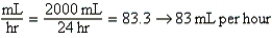# Directions: Calculate the Iv Flow Rates with Da Equations Where

Question 2
Essay

## Directions: Calculate the IV flow rates with DA equations where requested.For credit,show work.Label all answers.Label flow rates in milliliters per hour or drops per minutes,as needed. Ordered: LRS 2 L IV q24h. a.Is this an intermittent or continuous order? What flow rate will the nurse set on an infusion pump? b.DA equation: c.Evaluation:

a.Continuous b.c.Equation is balanced.mL per hour remains.